# 博客

Tags
None

## 非官方省队互测 Online Judge is running

2023-06-06 17:21:53 By Qingyu

whqsing。

## QOJ 常见问题（Q&A）

2023-03-23 22:14:28 By Qingyu

#### 什么是 QOJ？QOJ 由谁在维护？

QOJ 是由我（Qingyu）搭建、开发并维护的在线评测系统（Online Judge）。QOJ 希望整理、分类并维护世界各地的算法竞赛题目、比赛信息与排行榜等数据，并为所有人提供题目练习或模拟训练的平台。

QOJ 的系统、题目与比赛的维护工作均主要由我进行。同时几位其他管理员会辅助参与 QOJ 的管理工作，他们分别是 flower, alpha1022Qiuly。感谢他们愿意抽出宝贵的时间来参与到 QOJ 的管理工作当中。

QOJ 基于开源项目 Universal OJUniversal OJ 社区版 进行二次开发，没有 vfk 与开源社区的劳动成果 QOJ 将不会存在，在此感谢整个 UOJ 开源社区对 UOJ 的开发与维护。

#### QOJ 的编译器信息是什么？

• Python2: Python 2.7.18
• Python 会先编译为优化过的字节码.pyo文件。
• Python3: Python 3.10.9
• Python 会先编译为优化过的字节码.pyo文件。
• C/C++: GCC 11.1.0
• 编译命令为 g++ code.cpp -o code -lm -Ofast -DONLINE_JUDGE
• 选择对应语言版本时会增加 -std=c++??
• Java 8/Java 11: openjdk version "11.0.16"
• 编译命令为 javac code.java
• Pascal: fpc 3.0.4+dfsg-23
• 编译命令为 fpc code.pas -O2

#### QOJ 的评测机 CPU 是什么？

Intel(R) Xeon(R) Platinum 8168 CPU @ 2.70GHz

#### QOJ 的特殊题目标签含义是什么？

• Interactive：这是一道交互题。选手可能会使用 I/O 或其他方式与交互库进行交互。
• Output Only：这是一道提交答案题。选手不需要提交代码文件，只需要上传答案文件。
• Communication：这是一道通信题。选手可能需要提交多个程序，或同一个程序会被运行多次。
• Unavailable：题目不可用。可能是我们没有对应题目的数据或辅助测评程序，也可能是我们的硬件资源无法满足题目测评要求（例如在 100 个线程中并行评测的题目）。

#### 我不想公开我的提交记录，有没有什么方法？

1. 你在一场允许使用 Hack 功能的比赛当中提交了这份代码，且对方是对应比赛的参赛选手。
2. 你在一场比赛中提交了这份代码，且对方是对应比赛的管理员。
3. 对方是对应题目的管理员。

#### QOJ 的 ICPC 赛制有没有注意事项？

QOJ 的 ICPC 赛制将在最后一小时进行封榜，最后一小时其他队伍提交的结果将在比赛完成后可见。

#### 在什么情况下，我参加比赛会被计算 Rating？

1. 你是对应比赛的正式选手，被移入非正式选手（用户名前带有星号）或 VP 参加的选手不会被计入 Rating。
2. 你满足对应比赛的计分要求：
• （OI 赛制）：你提交了至少一份代码，且得到了非零的分数。
• （IOI 赛制）：你提交了至少一份代码，且得到了非零的分数。
• （ICPC 赛制）：你提交了至少一份代码，通过了对应题目的样例数据。

#### 我在使用 QOJ 的过程中出现了问题，能不能联系你们？

1. 站内私信 Qingyu
2. 发送邮件至 [email protected]

## Public CTS Round #1 题解

2023-01-07 10:16:08 By Qingyu
• 组题人：Qingyu
• 搬题人：
• 黑白点：flower, Qingyu
• 博弈：Qingyu
• 地雷：flower, p_b_p_b
• 桥桥桥：Qingyu
• 游戏：Qingyu
• 知识：alpha1022, Qingyu
• 题解：flower, Qingyu, LeafSeek, alpha1022

Source:

### 博弈

Source: Кубок трёх четвертьфиналов 2019. Subregional 1. Moscow.

### 地雷

#### 算法3

1. 我们在 $rt$ 处理爆炸路径经过 $rt$ 的点对 $(u, v)$，这意味着 $(u, v)$ 可能在 $rt$ 为根的同一子树。
2. 我们仍然在 $rt$ 处计算所有跨过$rt$ 的 $(u, v)$ 能不能到达，但是为此我们需要预处理处一些连通块外的信息。

### 桥桥桥

• 取出 $G$ 的任意一棵生成树 $T$
• 对于所有非树边 $e$，随机一个 $[0, 2^{64})$ 内的权值 $w(e)$
• 对于所有树边 $e$，定义其权值 $w(e)$ 为所有覆盖它的非树边的权值的异或和。

• 选择了 $0$ 条树边，$3$ 条非树边。
• 选择了 $1$ 条树边，$2$ 条非树边。
• 选择了 $2$ 条树边，$1$ 条非树边。
• 选择了 $3$ 条树边，$0$ 条非树边。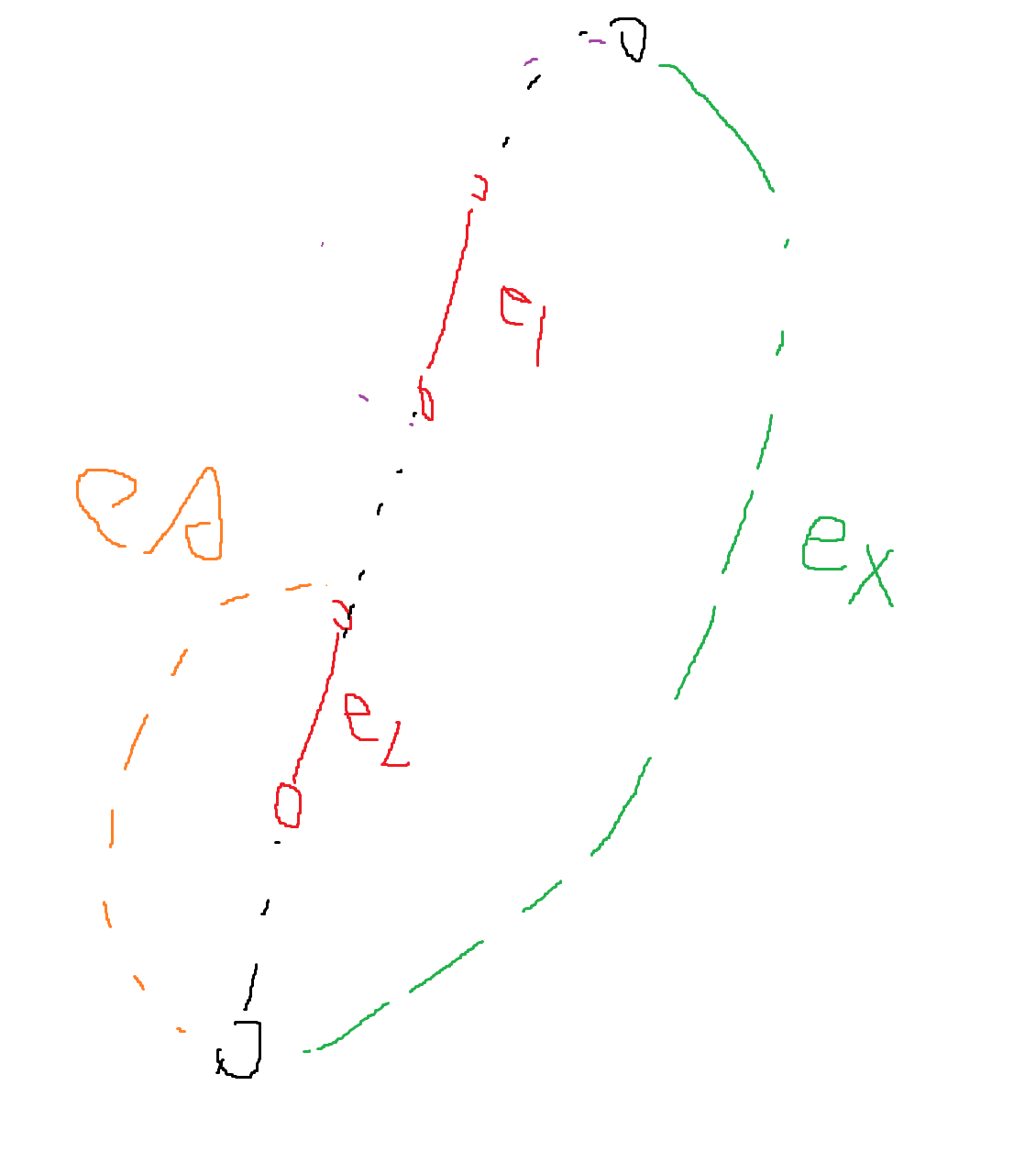### 游戏

Source:

#### 算法一

1. 如果不删空连续段，则这次操作可能会让这个 2 变为 12
2. 否则连续段被删空，如果 2 处于开头或结尾，则 2 消失。
3. 否则 2 处于中间，2 会被删除且前后的元素会被合并，即 ?2? 会被转化为 2

by LeafSeek

### 知识

Source:

$$L_{ij} = \begin{cases} \sum_{(k,i) \in E} w_{ki}, & i=j \\ -w_{ij}, & (i,j) \in E \\ 0, & \text{otherwise} \end{cases}$$

$$A \otimes B = \begin{bmatrix} a_{11} B & a_{12} B & \cdots & a_{1m} B \\ a_{21} B & a_{22} B & \cdots & a_{2m} B \\ \vdots & \vdots & \ddots & \vdots \\ a_{n1} B & a_{n2} B & \cdots & a_{nm} B \end{bmatrix}$$

 潘佳奇，浅谈线性代数与图论的关系，IOI 2021 中国国家集训队论文

## QOJ 更新日志（2023 Sep）

2023-01-06 20:27:29 By Qingyu

### 09/29/2023

1. 添加对 Rust 的支持，采用的编译器版本为 rustc 1.66.1，编译选项为 rustc -o answer -C opt-level=3 answer.code
2. 将提交记录的代码高亮库更换为 highlight.js

### 09/11/2023

1. 主站的评测机数更改为 5 台。每台评测机的 CPU 均为 Intel(R) Xeon(R) Platinum 8370C CPU @ 2.80GHz。其中评测机 #1 的内存为 32 GiB，其余两台评测机的内存为 16 GiB。
2. 修改了沙盒中的部分实现。由于此前编写的 judger 有部分不兼容新的沙盒，因此目前所有使用了自定义 judger 的题目均被暂时隐藏。他们将在确认兼容性后重新公开。

### 08/28/2023

1. 增加了对可任意选择 Time Window 开始时间赛制的支持。
2. 增加了对包含多个固定的 Time Window 的赛制的支持。
3. 增加比赛管理员可以为某个用户单独延长比赛时间的特性。
4. 比赛管理员现在可以取消用户的报名。
5. 修复了在 Time Window 结束后的提交仍然会被算入比赛提交的 bug。
6. 不再禁止用户选择开始时间比当前时间更早的 Time Window。
7. 比赛结束后的提交现在也可以在比赛提交记录中查看。

### 08/22/2023

1. 主站的评测机数更改为 3 台。每台评测机的 CPU 均为 Intel(R) Xeon(R) Platinum 8370C CPU @ 2.80GHz。其中评测机 #1 的内存为 32 GiB，其余两台评测机的内存为 16 GiB。

### 08/10/2023

1. QOJ 开始记录评测记录的历史信息！

### 08/04/2023

1. 在注册 Virtual Contests 时会弹出确认列表。
2. 修复了在 VP 时可以查看题目统计信息的 bug。
3. 修复了在 IOI 赛制的 VP 时可以查看测试点详细信息的 bug。

### 04/27/2023

1. 在 VP 时 Attachments 会默认隐藏题解。
2. 在 VP 时禁止查看比赛中其他人的提交记录。

### 03/21/2023

Category 增加显示每个子类别比赛与题目数。

### 03/04/2023

1. 对应比赛的管理员可设置在比赛进行时排行榜的显示范围（是否显示正式选手/Virtual 选手，以及每类 Ghosts 的范围）
2. 对应比赛的管理员可导出比赛的 Standings 文件（.dat / TestSys 格式）
3. 在导入 Polygon 格式的题目时，使用标准校验器的题目将默认配置 use_builtin_checker，而不再使用独立的 checker
4. 检验数据正确性不再要求配置 Main Correct Solution。

### 02/25/2023

1. 修复了无开始时间的比赛 VP 后不会滚榜的 bug。
2. 修复了 Category 对比赛排序时产生的问题。

### 02/03/2023

1. ICPC 赛制现支持设定封榜。
2. 修复了部分通信题出现 Dangerous Syscalls 的问题

### 01/09/2023

• Python2: Python 2.7.18
• Python3: Python 3.10.9
• C/C99/C11/C++/C++98/C++11/C++14/C++17/C++20/C++23: GCC 11.1.0
• Java 8/Java 11: openjdk version "11.0.16"
• Pascal: fpc 3.0.4+dfsg-23

## Public CTS Round #1 公告

2023-01-02 23:12:06 By Qingyu

## Public NOIP Round #4 题解

2022-11-20 15:28:33 By Qingyu
• 搬题人：
• 咖啡, 画图：Qingyu
• 治病, 拓扑序计数：feecle6418
• 序列：Wu_Ren
• 水果：p_b_p_b
• 组题人：Qingyu
• 题解：alpha1022, feecle6418, Wu_Ren, p_b_p_b

### 咖啡 (Div. 2 Only)

Tutorial by alpha1022.

### 画图 (Div. 2 Only)

Tutorial by alpha1022.

### 治病 (Div. 1 + Div. 2)

Tutorial by feecle6418.

### 拓扑序计数 (Div. 1 + Div. 2)

Tutorial by feecle6418.

for i from n-1 downto 0:
for j from 2^i to (2^{i+1}-1):
ans[i]+=a[j]
a[j-2^i]+=a[j]

### 序列 (Div. 1 Only)

Tutorial by Wu_Ren.

$mx,sz$ 的合并是显然的。考虑 $len$ 的合并，首先，假如合法子段不经过 $u$，那么就是 $len_u\leftarrow \max(len_{lc},len_{rc})$。假设存在 $u\in[l,r]$ 使得 $[l,r]$ 是合法子段，那么假设 $\max\limits_{i\in[l,u-1]} \{a_i\}\ge \max\limits_{i\in[u+1,r]} \{a_i\}$，此时我们可以发现，如果 $l>u-sz_{lc}\land r>u$，那么 $[l-1,r-1]$ 也是合法的。

### 水果 (Div. 1 Only)

Tutorial by p_b_p_b.

• 如果 $a_x\ne -1$ ，那么有两种选择
• 让 $a_x$ 成为最终的前缀最大值，那么从 $dp_{x-1,\lfloor a_x\rfloor}+c_{a_x}$ 转移。
• 扔掉 $a_x$ ，从 $dp_{x-1,v}$ 转移。
• 如果 $a_x=-1$ ，再分两种
• $a_1,\cdots,a_{x-1}$ 的最大值小于 $v$ ，那么这里肯定应该贪心放 $v$ ，从 $dp_{x-1,v-1}+c_v$ 转移。
• 否则这里只能被迫开摆，随便放个以后不用的小的，从 $dp_{x-1,v}$ 转移。（注意因为我们做过预处理，所以这里总是可以保证不超过 $v$ 。）

• 对于 $a_x\ne -1$ ，注意到 $dp_{x,0},\cdots,dp_{x,m}$ 单调不降，因此一定是一个前缀从 $dp_{x-1,\lfloor a_x\rfloor}+c_{a_x}$ 转移，而其他地方直接把 $dp_{x-1,v}$ 拉过来。因此只要二分分界点就可以直接前缀赋值。
• 对于 $a_x=-1$ ，显然只有一个合法状态会从 $dp_{x-1,v}$ 转移，剩下的都从 $dp_{x-1,v-1}+c_v$ 转移。不过这个怎么维护呢？

## Public NOIP Round #4 公告

2022-11-13 02:21:21 By Qingyu

Public NOIP Round #4 将在 2022 年 11 月 20 日 8:30 举行！

## Public NOIP Round #3 题解

2022-10-22 14:38:11 By Qingyu
• 搬题人：
• 数字：gyh20
• 因子：Wu_Ren
• 移除石子：feecle6418
• 抓内鬼：p_b_p_b
• 异或序列：feecle6418
• 数圈圈：feecle6418
• 组题人：hehezhou
• 题解：gyh20, Wu_Ren, feecle6418, p_b_p_b

### 数字 (Div. 2 Only)

Tutorial by gyh20.

Submission #56492 - Public Judge (pjudge.ac)

Submission #56801 - Public Judge (pjudge.ac)

### 因子 (Div. 2 Only)

• Petrozavodsk Summer 2020. Day 5: JAG Summer+ Opening Contest. Problem B. Non-Trivial Common Divisor
• ACM-ICPC Japan Alumni Group Summer Camp 2019. Problem B. Non-Trivial Common Divisor
• https://qoj.ac/contest/505/problem/1336

Tutorial by Wu_Ren.

### 移除石子 (Div. 1 + Div. 2)

Tutorial by feecle6418.

#### 算法二

1. $X,Y$ 都是横坐标最大的点，这时靠右放个正方形，可以删掉 $X,Y$。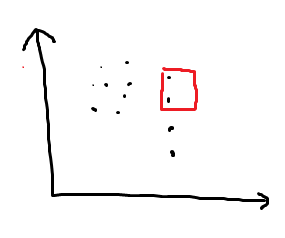2. 横坐标最大的点只有 $X$。

1. $Y$ 的纵坐标小于或等于 $X$ 的纵坐标，则放一个以 $Y$ 为左下顶点的很大的正方形。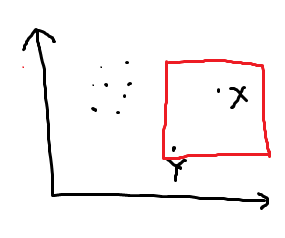2. $Y$ 的纵坐标大于 $X$ 的纵坐标。

1. $Z$ 的横坐标等于 $Y$ 的横坐标，$Z$ 的纵坐标大于 $X$ 的纵坐标。

这时以 $YZ$ 为一边放一个正方形就可以删掉 $Y,Z$：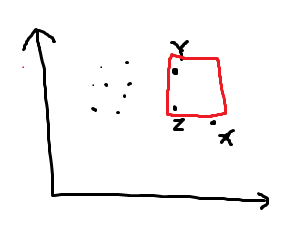2. $Z$ 的横坐标等于 $Y$ 的横坐标，$Z$ 的纵坐标恰好等于 $X$ 的横坐标。

这时以 $YZ,ZX$ 中短的一边作为正方形的一条边，比如下图中 $YZ\le ZX$，就这样画：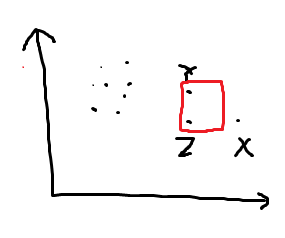注意，为了防止 $YZ=ZX$ 时把 $XYZ$ 三个点恰好都框住，需要把正方形偏移 0.5 个单位，这也是题目允许输出小数的原因。

3. 不是以上两种情况，则以 $(x_Y,y_X)$ 为左下角作一个很大的正方形即可：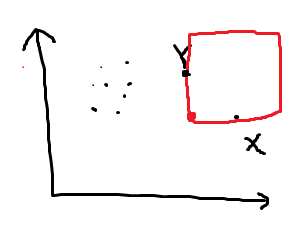### 抓内鬼 (Div. 1 + Div. 2)

• Noric Collegiate Programming Contest 2021. Problem C. Customs Control
• Petrozavodsk Winter 2022. Day 4: Almost Northern Contest. Problem C. Customs Control
• https://qoj.ac/contest/822/problem/1774

Tutorial by p_b_p_b.

#### 算法一

$k=1$ 。

#### 算法二

$u_i\in \{1,n\}$ 或 $v_i\in \{1,n\}$ 。

### 异或序列 (Div. 1 Only)

• Petrozavodsk Winter 2021. Day 8: Belarusian SU Contest, Yandex Cup 2021. Problem C. Brave Seekers of Unicorns
• XXI Open Cup named after E.V. Pankratiev, Grand Prix of Belarus. Problem C. Brave Seekers of Unicorns
• https://qoj.ac/contest/536/problem/1085

Tutorial by feecle6418.

### 数圈圈 (Div. 1 Only)

• Petrozavodsk Winter 2021. Day 8: Belarusian SU Contest, Yandex Cup 2021. Problem F. Border Similarity Undertaking
• XXI Open Cup named after E.V. Pankratiev, Grand Prix of Belarus. Problem F. Border Similarity Undertaking
• https://qoj.ac/contest/536/problem/1088

Tutorial by feecle6418.

#### 算法四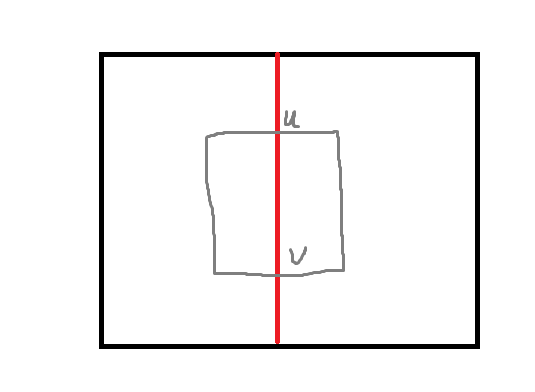## Public NOIP Round #2 题解

2022-10-04 13:34:02 By Qingyu
• 搬题人：
• 就这：Y25t
• 保序回归问题：Y25t
• 恰钱：skip2004
• 排序：Wu_Ren
• 图同构：hehezhou
• 找零：p_b_p_b
• 组题人：p_b_p_b
• 题解：p_b_p_b, Wu_Ren, Y25t, nantf

### 就这 (Div. 2 Only)

• 2021-2022 ICPC Northern Eurasia - Belarus Regional Contest. Problem A. Constructiveforces
• Кубок Трёх Четвертьфиналов 2021. Problem A. Constructiveforces

Tutorial by Y25t

for(int i=0;i<n;i++) std::cout<<(i%m<k);

### 保序回归问题 (Div. 2 Only)

• 2021-2022 ICPC Northern Eurasia - Belarus Regional Contest. Problem E. Positive Thinking
• Кубок Трёх Четвертьфиналов 2021. Problem E. Positive Thinking

Tutorial by Y25t

### 恰钱：(Div. 1 + Div. 2)

• The 2022 ICPC Asia Regionals Online Contest (I)

Tutorial by p_b_p_b

• 当 $\text{ctz}(x)\le 5$ 时 $\text{ppc}(x)$ 也很小，这样只会有 $O((\log r)^4)$ 个合法的 $x$ 。
• 否则这样的 $x$ 只有 $r/2^5$ 个，也不会太多，把合法的留下就更少了。

### 排序：(Div. 1 + Div. 2)

• Petrozavodsk Winter 2020. Day 8. Almost Algorithmic Contest. Problem C. StalinSort Algorithm

tutorial by Wu_Ren

### 图同构：(Div. 1 Only)

• 2021“MINIEYE杯”中国大学生算法设计超级联赛（6）. Problem 1007. Power Station of Art
• Petrozavodsk Summer 2021. Day 6. Xi'an JTU Contest 1, Grand Prix of Xi'an. Problem G. Power Station of Art
• XXII Open Cup named after E.V. Pankratiev, Grand Prix of Xi'an. Problem G. Power Station of Art

Tutorial by nantf

1. 该连通块为二分图

2. 该连通块不为二分图

• 将 $x$ 上的二元组沿反方向交换一圈到 $y$ 点，然后将原本 $y$ 上的二元组沿反方向交换一圈到 $x$ 点。

### 找零：(Div. 1 Only)

• 京都大学プログラミングProgrammingコンテストContest 2021. Problem F. One Yen Coin
• Petrozavodsk Winter 2022. Day 1. Kyoto U Contest 2. Problem F. Flatland Currency.
• XXII Open Cup named after E.V. Pankratiev, Grand Prix of Kyoto. Flatland Currency.

Tutorial by p_b_p_b

• 注意到如果价值相同，那么一定是按价格从小到大选。所以可以设 $dp_{i,j}$ 表示考虑了所有价值 $\le i$ 的物品，获得的价值为 $j$ ，所需的最小代价，然后利用决策单调性在 $O(n\log n)$ 的时间内从 $dp_i$ 转移到 $dp_{i+1}$ 。
• 注意到价值的 $\text{lcm}$ 是 $12$ ，所以可以把每种价值的物品分别先打包成价值为 $12$ 的包裹，然后按照价格从小到大选包裹。这样的一个问题是最优解的价值并不一定是 $12$ 的倍数，但是可以枚举价值为 $1,2,3,4$ 的物品选的个数模 $12,6,4,3$ 的值，然后先把这些物品选掉之后再贪心即可。
• 放弃分析，直接先按照性价比排序贪心选，然后在每种价值物品的边界附近进行小背包。大概和前一种是等价的。

## Public Easy Round #3 题解

2022-09-22 16:44:28 By Qingyu
• 搬题人：
• DNA 匹配 2：Qingyu
• 情报传递 3：Qingyu
• 别急 2：Qingyu
• 旅程：flower
• 染色：Qingyu
• 运算符 2：feecle6418
• 匹配求和：Qingyu
• 组题人：Qingyu
• 验题人：feecle6418, flower, gyh20, test12345
UOJ 缺投！

### DNA 匹配 2（50 Points）

• infO(1) Cup 2017 National Round. Problem 2, DNA

### 情报传递 3（50 Points）

• ByteDance-Moscow Workshops Camp 2022. Shuffle Contest. Problem M, Multiple Communications

### 别急 2（75 Points）

• ByteDance-Moscow Workshops Camp 2022. Shuffle Contest. Problem B, Broken Connection

### 旅程（75 Points）

• Natjecanje timova studenata informatičara hrvatskih sveučilišta 2012. Problem G. Restorani

#### 算法 1

1. 不经过这个 scc，代价是 0。
2. 经过 $k$ 个此 scc 的点，其中 $1$ 个点的代价 $y_u$，剩下 $k-1$ 个点的代价是 $x_u$。

### 运算符 2（125 Points）

• Petrozavodsk Winter 2021. Day 8. Belarusian SU Contest, Yandex Cup. Problem A. Belarusian State University
• XXI Open Cup named after E.V. Pankratiev, Grand Prix of Belarus. Problem A. Belarusian State University

#### 算法 1

• 恒 0 / 恒 1。这时可以通过重新对下标赋值，将其转为 AND 运算。
• $f(x,y)=x$ 或 $f(x,y)=y$，或 $f(x,y)$ 等于 $x$ 或 $y$ 取反。这时可以通过重新对无关一边的下标赋值 1，将其转为 AND 运算。
• 等价于，或把 $x$ 取反后等价，或把 $y$ 取反后等价，或均取反后等价 AND/XOR。

### 匹配求和（200 Points）

• Petrozavodsk Winter 2020. Day 9. Yuhao Du Contest 7. Problem F. Fast as Ryser
• XX Open Cup named after E.V. Pankratiev, Grand Prix of Zhejiang. Problem F. Fast as Ryser

#### 算法 1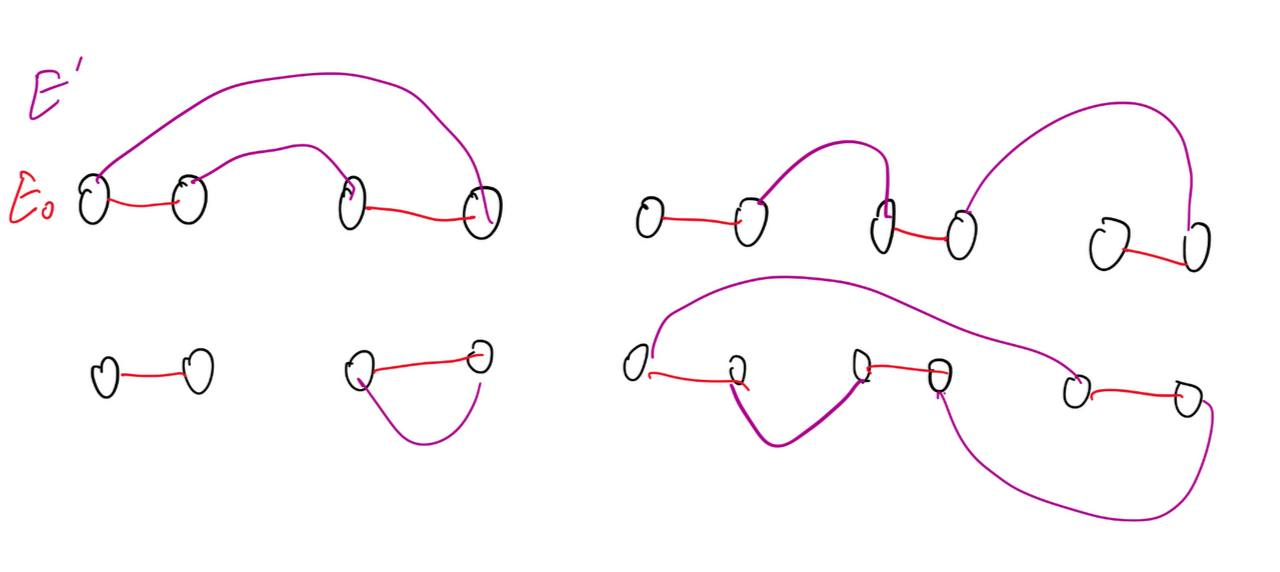UOJ 缺投！

• 1
• 2
• 3
• 4